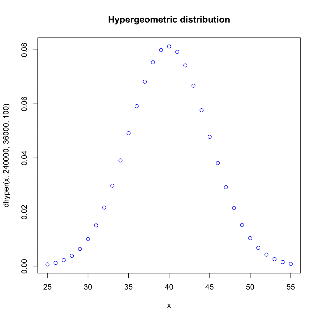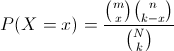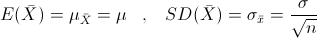## Monday, February 21, 2011

### Using R for Introductory Statistics, Chapter 5, hypergeometric distribution

This is a little digression from Chapter 5 of Using R for Introductory Statistics that led me to the hypergeometric distribution.Question 5.13 A sample of 100 people is drawn from a population of 600,000. If it is known that 40% of the population has a specific attribute, what is the probability that 35 or fewer in the sample have that attribute.

I'm pretty sure that you're supposed to reason that 600,000 is sufficiently large that the draws from the population are close enough to independent. The answer is then computed like so:

```> pbinom(35,100,0.4)
 0.1794694
```

Although this is close enough for practical purposes, the real way to answer this question is with the hypergeometric distribution.

The hypergeometric distribution is a discrete probability distribution that describes the number of successes in a sequence of k draws from a finite population without replacement, just as the binomial distribution describes the number of successes for draws with replacement.

The situation is usually described in terms of balls and urns. There are N balls in an urn, m white balls and n black balls. We draw k balls without replacement. X represents the number of white balls drawn.R gives us the function phyper(x, m, n, k, lower.tail = TRUE, log.p = FALSE), which does indeed show that our approximation was close enough.

```> phyper(35,240000,360000, 100)
 0.1794489
```

Since we're down with OCD, let's explore a bit further. First, since our population is defined and not too huge, let's just try it empirically. First, create our population.

```> pop <- rep(c(0,1),c(360000, 240000))
> length(pop)
 600000
> mean(pop)
 0.4
> sd(pop)
 0.4898984
```

Next, generate a boatload of samples and see how many of them have 35 or fewer of the special members.

```> sums <- sapply(1:200000, function(x) { sum(sample(pop,100))})
> sum(sums <= 35) / 200000
 0.17935
```

Pretty close to our computed results. I thought I might be able to compute an answer using the central limit theorem, using the distribution of sample means, which should be approximately normal.```> means <- sapply(1:2000, function(x) { mean(sample(pop,100))})
> mean(means)
 0.40154
> sd(means)
 0.0479998
> curve(dnorm(x, 0.4, sd(pop)/sqrt(100)), 0.2, 0.6, col='blue')
> lines(density(means), lty=2)
```Shouldn't I be able to compute how many of my samples will have 35 or fewer special members? This seems to be a ways off, but I don't know why. Maybe it's just the error due to approximating a discreet distribution with a continuous one?

```> pnorm(0.35, 0.4, sd(pop)/sqrt(100))
 0.1537173
```

This fudge gets us closer, but still not as close as our initial approximation.

```> pnorm(0.355, 0.4, sd(pop)/sqrt(100))
 0.1791634
```

If anyone knows what's up with this, that's what comments are for. Help me out.

#### Notes on Using R for Introductory Statistics

Chapters 1 and 2

Chapter 3

Chapter 4

Chapter 5

1.The 'fudge' is your continuity correction.

And 0.00028 is close enough for the approximation, there is nothing wrong. That's about 0.15% absolute error.

2.Cool, thanks!

3.Sorry I meant to say 0.15% relative error.